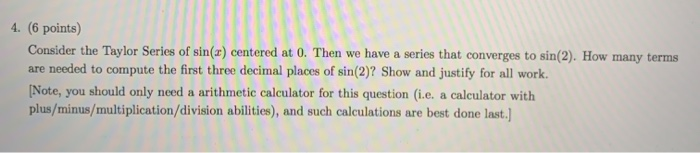# Question 4. (6 points) Consider the Taylor Series of sin(x) centered at 0. Then we have a series that converges to sin(2). How many terms are needed to compute the first three decimal places of sin(2) Show and justify for all work ! (Note, you should only need a arithmetic calculator for this question (i.e. a calculator with plus/minus/multiplication/division abilities), and such calculations are best done last.) i